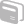# Activities

•• ##### Subject Area

• Math: Algebra II: Equations
• Math: Algebra II: Systems of Linear Equations and Inequalities
• Math: Algebra I: Equations and Inequalities
• Math: Algebra I: Linear Functions

• ##### Author9-12

45 Minutes

• ##### Device
• TI-83 Plus Family
• TI-84 Plus
• TI-84 Plus Silver Edition
• TI-Navigator™
• ##### Software

LearningCheck™ Creator

## Writing and Solving Systems of Equations Using Cabri Jr.

#### Activity Overview

Through this introduction to the use of the Cabri Jr. application in the Algebra classroom, students will algebraically and graphically determine equations of lines, as well as determine solutions to systems of linear equations. Using Cabri Jr's dynamic, transformational features, students will also create and solve multiple systems of linear equations, including those involving parallel and perpendicular lines.

#### Before the Activity

This lesson is designed as an introduction to the use of Cabri Jr. in the Algebra I or Algebra II class. Students should first work through the handout (which teachers may want to print on separate pages so that students can algebraically determine solutions before they use Cabri Jr. to check their solutions to equations of lines and solutions of systems). After students work through the handout, the Learning Check can be used to determine mastery of the material. (The solution to Learning Check quiz question #4 assumes that vertical, parallel lines have undefined slopes.)

#### During the Activity

Through this introduction to the use of the Cabri Jr. application in the Algebra classroom, students will algebraically and graphically determine equations of lines, as well as determine solutions to systems of linear equations. Using Cabri Jr's dynamic, transformational features, students will also create and solve multiple systems of linear equations, including those involving parallel and perpendicular lines.